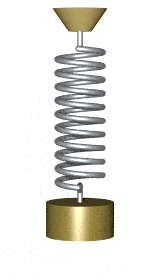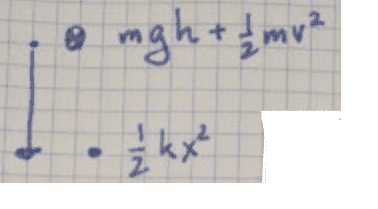# Find v(x) of a mass suspended from a spring

Homework Statement:
A mass (m=0.2 kg) is suspended from a spring (D/k=10 N/m)

When x = 0 (Spring is relaxed, at equilibrium), v(0) is 3 m/s.

Find v(x) as a first order differential equation.
Relevant Equations:
I tried using energy conservation to check my work, but I think I messed up. I boxed in my solution. Above is me trying to verify with the conservation of energy and below is my attempt.
Hi all, I have a problem that I've been grappling with for the past 2 hours.

I was confident at first that I found the correct solution, but when I tried to verify I didn't have a constant in my v(x) function.

Here is my attempt:
I appreciate your help kind internet strangers!

BvU
Homework Helper
I boxed in my solution

What also throws me off is that they gave me k and m, but I didn't need it for my solutionI'm sorry, I don't understand?

BvU
Homework Helper

Do you get a cookie notification from imgur? Meaning I should repost my picture?

#### Attachments

•IMG_20190603_172630.jpg
85.8 KB · Views: 175
BvU
Homework Helper
Don't know what went wrong:BvU
Homework Helper
What also throws me off is that they gave me k and m, but I didn't need it for my solutionYou need ##k## and ##m## in the differential equation you are supposed to set up -- at least according to your own problem statement:
Find v(x) as a first order differential equation.

•spsch
haruspex
Homework Helper
Gold Member
2020 Award
didn't have a constant in my v(x) function.
Please clarify: which equation and which constant do you think you are missing?
If you mean your differential equation (the one with vdv/dx) and the constant C, you would not expect that constant in that equation. The given information about the value of v at x=0 is redundant.

•spsch
Please clarify: which equation and which constant do you think you are missing?
If you mean your differential equation (the one with vdv/dx) and the constant C, you would not expect that constant in that equation. The given information about the value of v at x=0 is redundant.
Hi, can you see my attachement https://www.physicsforums.com/attachments/img_20190603_172630-jpg.244546/ (i am asking because I seem not to be able to open all)

When I solve for v(x) with differential equations and when I use conservation of Energy I get two solutions that differ by a constant 9 under the square root.

The function itself is also weird shouldn't v(x) eventually come to 0 when kx = mg?

haruspex
Homework Helper
Gold Member
2020 Award
When I solve for v(x) with differential equations and when I use conservation of Energy I get two solutions that differ by a constant 9 under the square root.
I was misled by the layout of your working. I thought you were comparing the boxed equation half way down with the final one.
Right at the start I see you have ##\frac 12kx^2=mgh+\frac 12mv^2##.
Please explain the basis of this equation and what h represents there.
shouldn't v(x) eventually come to 0 when kx = mg?
Why? If that condition arises it would then be static.

•spsch
I was misled by the layout of your working. I thought you were comparing the boxed equation half way down with the final one.
Right at the start I see you have ##\frac 12kx^2=mgh+\frac 12mv^2##.
Please explain the basis of this equation and what h represents there.

Why? If that condition arises it would then be static.
Hi Haruspex.
Yes, I'm sorry the layout is bad I see that now. I had the work on another sheet and I actually thought it would be more clear this way.

The above is me trying to verify my solution by using conservation of energy.

This is what I thought:
At the middle where v is at it's maximum and x = 0 there is only potential energy mgh (or mgy) and the kinetic energy which is at it's maximum there.
On the way down the spring force works against the kinetic energy and gravity, i.e. pulls the mass up.

if we say that h= 0 where kx = mg then all the energy there, at the bottom so to say is(1/2)*k*x^2
##\frac 12kx^2##. So all of the potential energy and kinetic energy turned into potential spring energy.

I dropped the minus sign because g is negative (-)(-) = (+), but I've wondered about whether I made a mistake here myself already, I actually dreamt about it.

I thought at the bottom v should be zero for a short time and reverse. I was imagining it.

BvU
Homework Helper
I thought at the bottom v should be zero for a short time and reverse. I was imagining it
I don't think you imagine it:(picture from Wikipedia)

Please confirm that you are searching for a first-order differential equation for ##v##, as your post #1 indicated...

(because usually we deal with this harmonic oscillator as a second-order differential equation in ##x##)

•spsch
Hi BvU

This is exactly what I was imagining it to look like (your gif animation).

I am looking for first-order differential equation.

The exact problem is two parts, a and b.

a) Find a 1st Order differential Equation Expression for v(x).

b) Solve the Equation you found for v(x)

it's from an old swiss high school physics exam.

haruspex
Homework Helper
Gold Member
2020 Award
At the middle where v is at it's maximum and x = 0
There is an apparent conflict in the given information. It says "When x = 0 (Spring is relaxed, at equilibrium)", but the equilibrium position (with the mass attached) is not with the spring relaxed.
Anyway, I'll assume it means equilibrium.
But your energy equation is still wrong. The total energy is constant. Rewrite the equation to express that.

There is an apparent conflict in the given information. It says "When x = 0 (Spring is relaxed, at equilibrium)", but the equilibrium position (with the mass attached) is not with the spring relaxed.
Anyway, I'll assume it means equilibrium.
But your energy equation is still wrong. The total energy is constant. Rewrite the equation to express that.
Hi Haruspex,
I am sorry!
It actually only says at x=0 where the spring is relaxed v = 3 m/s. Assumed that this must be equilibrium and attempted to translate.

There is a diagram with it. I attached a screenshot.

I will redo the equation and see if I can get the two to mean the same thing!

thank you very much.

there also is a hint with a = v*dv/dx, which is why I went with kx-mg=mv*dv/dx

#### Attachments

haruspex
Homework Helper
Gold Member
2020 Award
It actually only says at x=0 where the spring is relaxed v = 3 m/s.
Ok, but try to write the energy conservation equation now.

•spsch
Ok, but try to write the energy conservation equation now.
Thank you, I am, I'm trying to figure out where I'm implying that it isn't constant. I had thought of the way that I wrote it that it expresses that the total energy is constant.
:-S.

haruspex
Homework Helper
Gold Member
2020 Award
Thank you, I am, I'm trying to figure out where I'm implying that it isn't constant. I had thought of the way that I wrote it that it expresses that the total energy is constant.
:-S.
You have spring PE on one side and KE on the other, both positive. E.g. both can increase with no change to the GPE.

•spsch
You have spring PE on one side and KE on the other, both positive. E.g. both can increase with no change to the GPE.
Hello Haruspex,

Thank you very much for your help.
I gave it a lot of thought, and by myself, I still didn't quite get what's wrong.

But by your hint, I think I may have figured out what the right equation is.
I rewrote it and uploaded it as an attachment, together with a drawing which attempts to explain my thinking in how I got the first equation.
Would you mind checking if the new equation is correct?

I changed h to be A-x, where A is the amplitude.

I am sorry if I'm a bit slow, I haven't had formal schooling, and I found that I sometimes taught myself things the wrong way when it's way too late. But that's why I also really appreciate the help, like having a teacher!

#### Attachments

haruspex
Homework Helper
Gold Member
2020 Award
Hello Haruspex,

Thank you very much for your help.
I gave it a lot of thought, and by myself, I still didn't quite get what's wrong.

But by your hint, I think I may have figured out what the right equation is.
I rewrote it and uploaded it as an attachment, together with a drawing which attempts to explain my thinking in how I got the first equation.
Would you mind checking if the new equation is correct?

I changed h to be A-x, where A is the amplitude.

I am sorry if I'm a bit slow, I haven't had formal schooling, and I found that I sometimes taught myself things the wrong way when it's way too late. But that's why I also really appreciate the help, like having a teacher!
It's rather hard to follow your working without some explanation. Don't worry about the amplitude for now. That is a "boundary condition" we can plug in later if appropriate.
You are defining x as displacement from spring’s relaxed position. I'll assume up is positive.
At x, you have that the elastic PE (EPE) is ##\frac 12kx^2## and that the KE is ##\frac 12mv^2##
What is the GPE (relative to x=0)? So what expression do you get for the total energy at x?

Next, plug in the known values at x=0 to find the value of that constant energy.

•spsch
SammyS
Staff Emeritus
Homework Helper
Gold Member
Hi all, I have a problem that I've been grappling with for the past 2 hours.

I was confident at first that I found the correct solution, but when I tried to verify I didn't have a constant in my v(x) function.•